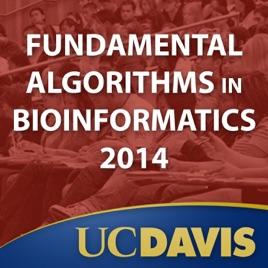32 episodios

This course covers fundamental algorithms for efficient analysis of biological sequences and for building evolutionary trees. This is an undergraduate course focusing on the ideas and concepts behind the most central algorithms in biological sequence analysis. Dynamic Programming, Alignment, Hidden Markov Models, Statistical Analysis are emphasized.# Fundamental Algorithms in Bioinformatics UC Davis

• Tecnología

This course covers fundamental algorithms for efficient analysis of biological sequences and for building evolutionary trees. This is an undergraduate course focusing on the ideas and concepts behind the most central algorithms in biological sequence analysis. Dynamic Programming, Alignment, Hidden Markov Models, Statistical Analysis are emphasized.

• video
Lecture 1: Introduction to bioinformatics and the course

## Lecture 1: Introduction to bioinformatics and the course

Introduction to the course and bioinformatics. Why we do bioinformatics, how it relates to genomics
and to the changing modalities of biology.

• 3 min
• video
Lecture 2: Further introduction

## Lecture 2: Further introduction

Continuation of the introduction to the course and
to bioinformatics. The biological utility of the similarity of sequences. The first fact of bio-sequence
analysis.

• 4 min
• video
Lecture 3: Defining sequence similarity

## Lecture 3: Defining sequence similarity

Definition of sequence similarity and string alignment,
counting alignments the need for fast computation.

• 4 min
• video
Lecture 4: Extending the model of sequence similarity

## Lecture 4: Extending the model of sequence similarity

Review of the definition of sequence similarity and
extensions of the model for greater biological fidelity.
Introduction to parametric sequence alignment.

• 4 min
• video
Lecture 5: Computing sequence similarity

## Lecture 5: Computing sequence similarity

Introduction to computational efficiency. Introduction
to how we actually compute sequence similarity efficiently.

• 3 min
• video
Lecture 6: Computing similarity using an alignment graph

## Lecture 6: Computing similarity using an alignment graph

Continuation of the discussion of how to efficiently
compute the similarity of two sequences. Introduction
to the traceback operation to find the
optimal alignment.

• 4 min